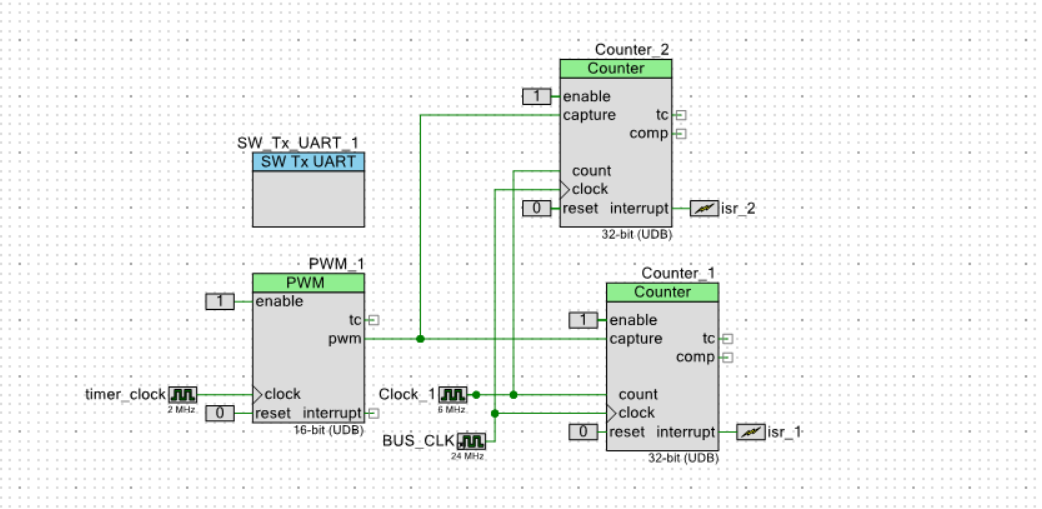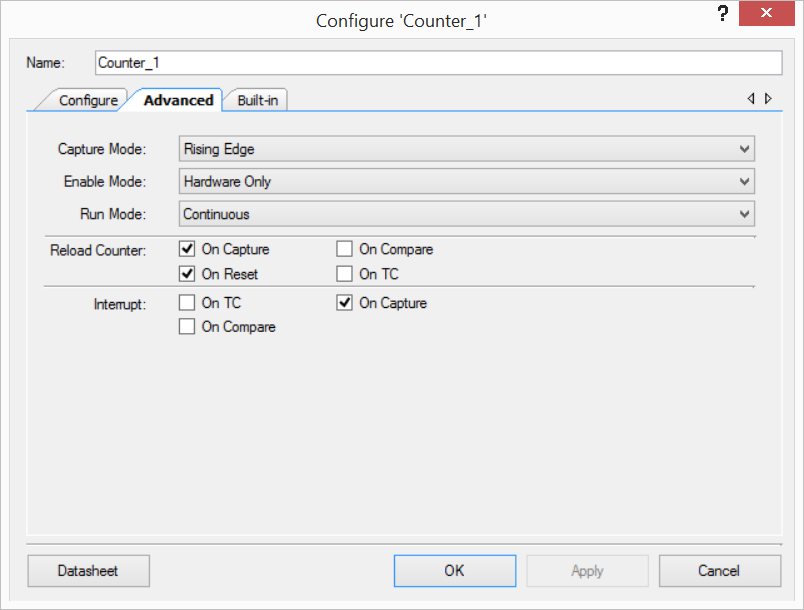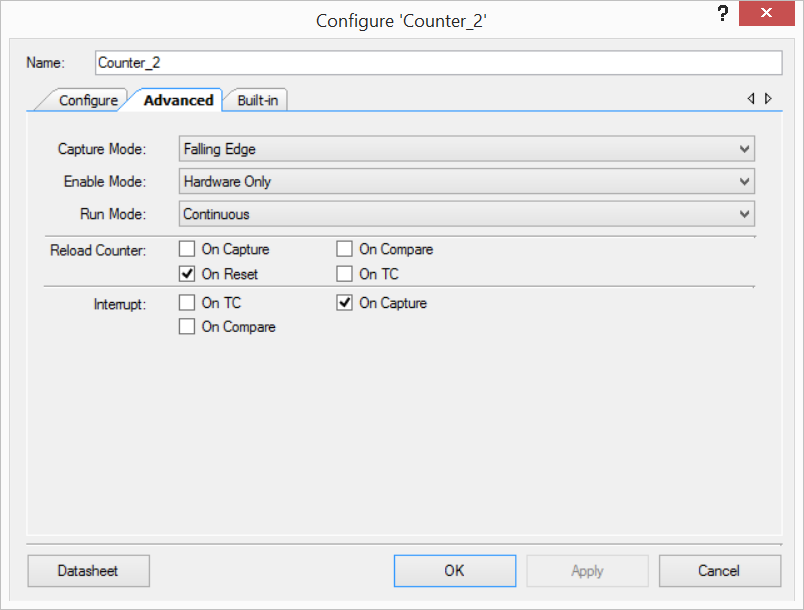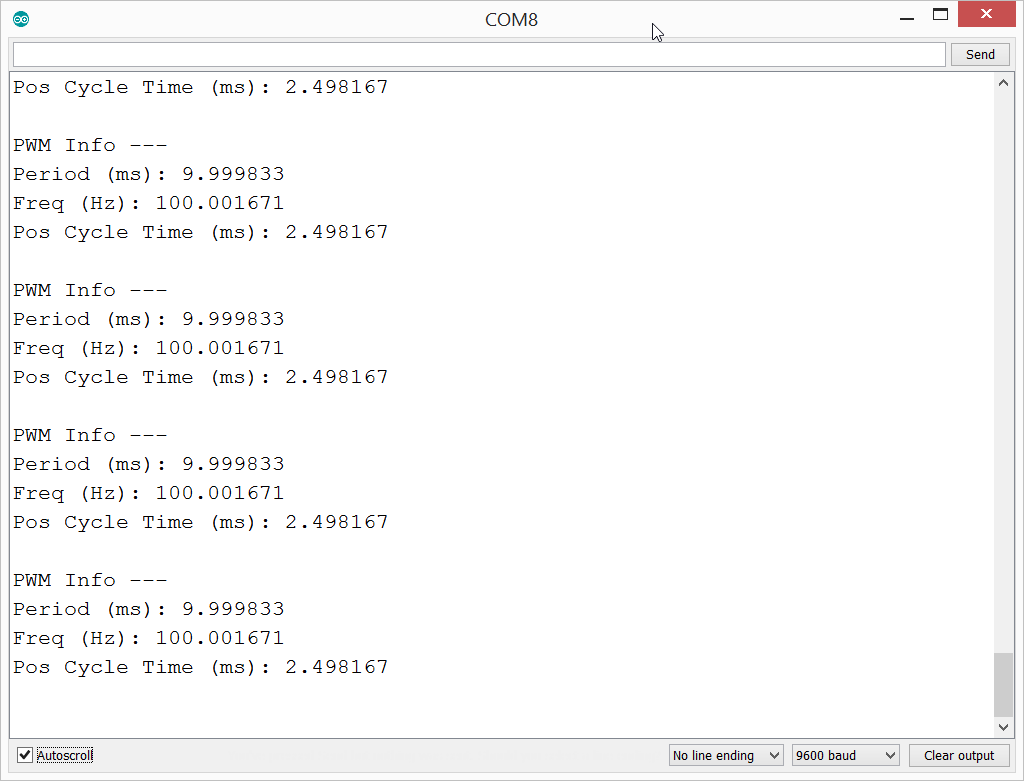Hello guys! In this post, we’ll learn how we can measure PWM values using a microcontroller/PSoC. Actually, I’m not sure about why we measure PWM, but I can predict something that, as you know some mechanical controllers like servo motors which can be controlled with PWM signals, so they have to analyse this signal and give the meaning of this signal.

Sometimes we need this method to understand what a PWM signal wants from us. So we measure this signal to get period, frequency and duty cycle values. Duty cycle is the most important value for controlling, after being sure the frequency of a PWM signal(it is like identity), we need to detect duty cycle value to decide speed etc.

Let’s look at the steps briefly (Algorithm);

1. Detect rising edges that follow each other (A): This time provides us the period value.

2. Detect rising edges and falling edges that follow each other (B): This time provides a value which we’ll use it to calculate the duty cycle.

3. Calculate the duty cycle value (C per cent): C = (B / A) * 100

Here are the steps for our PSoC 5LP device;

1. Add a PWM, two Counter components into the design.2. Set Counter_1 “Capture Mode” to “Rising Edge” and “Reload Counter” to “On Capture” and “On Reset”. Then set “Interrupt” to “On Capture”.3. Set Counter_2 “Capture Mode” to “Falling Edge” and “Reload Counter” to “On Reset” only.  Then set “Interrupt” to “On Capture”.4. Coding (My code is so complex/bad because I was ill that day, I slept as soon as I managed to complete. And I have no time to clear.):

4.1. When Counter_1 captures a rising edge, ISR is triggered. Get and store(A) Counter_1 count value and reset Counter_2 count value. (Counter_1 count value will be wrong in the first time)

4.2. When Counter_2 captures a falling edge, get Counter_2 count value and store(B).

4.3. Calculate C, it is equal to B/A

```#include "project.h"
#include "string.h"
#include "stdio.h"

float measuredTime=0u;
float measuredPosCycleTime=0u;
float measuredFreq=0u;

uint32 countOfEdgeForPeriod;
uint32 countOfEdgeForPosCycle;
uint8 counterCapture=0u;

#define freqClk (float)6000000u;
#define NO_OF_USEC (float)1000u

char period;
char posCycleTime;
char freq;

CY_ISR(rising_edge){
Counter_2_WriteCounter(0);
Counter_1_STATUS;
}

CY_ISR(falling_edge){
Counter_2_STATUS;
}

float calculatePeriod(uint32 count){
float time=0u;
time=(float)count / freqClk;
time*=NO_OF_USEC;
return time; //milliSec
}

float calculateFreq(float period){
float freq=0u;
freq=(float)1 / period;
return freq*1000; //Hz
}

int main(void)
{
isr_1_StartEx(rising_edge);
isr_2_StartEx(falling_edge);
CyGlobalIntEnable; /* Enable global interrupts. */
SW_Tx_UART_1_Start();
Clock_1_Start();
Counter_1_Start();
Counter_2_Start();
Counter_1_Enable();
timer_clock_Start();
PWM_1_Start();

SW_Tx_UART_1_PutString("hello\n");

for(;;)
{
measuredTime=calculatePeriod(countOfEdgeForPeriod);
measuredFreq=calculateFreq(measuredTime);
measuredPosCycleTime=calculatePeriod(countOfEdgeForPosCycle);
sprintf(period,"Period (ms): %f\n",measuredTime);
sprintf(freq,"Freq (Hz): %f\n",measuredFreq);
sprintf(posCycleTime,"Pos Cycle Time (ms): %f\n",measuredPosCycleTime);
SW_Tx_UART_1_PutString("PWM Info ---\n");
SW_Tx_UART_1_PutString(period);
SW_Tx_UART_1_PutString(freq);
SW_Tx_UART_1_PutString(posCycleTime);
SW_Tx_UART_1_PutChar('\n');
CyDelay(300);
}
}
```

Let’s see the result:Serial output

So the measurement of a PWM is not a difficult thing as we imagine it. If you measure for the first time, you can try to measure using Arduino and pulseIn method, I advise you to use Arduino for the first time.